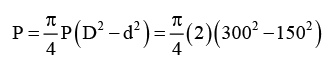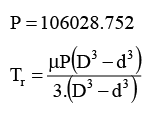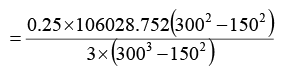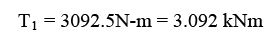Courses

# Machine Design - 2

## 20 Questions MCQ Test GATE Mechanical (ME) 2022 Mock Test Series | Machine Design - 2

Description
This mock test of Machine Design - 2 for GATE helps you for every GATE entrance exam. This contains 20 Multiple Choice Questions for GATE Machine Design - 2 (mcq) to study with solutions a complete question bank. The solved questions answers in this Machine Design - 2 quiz give you a good mix of easy questions and tough questions. GATE students definitely take this Machine Design - 2 exercise for a better result in the exam. You can find other Machine Design - 2 extra questions, long questions & short questions for GATE on EduRev as well by searching above.
QUESTION: 1

### A simply supported shaft of diameter 50 mm and length 2 m is supported to a load of 2.5 kN at its centre. The material of the shaft is brittle with an ultimate tensile strength of 300 MPa and theoretical stress concentration factor is 161. The maximum stress developed in the shaft is _________ MPa.

Solution: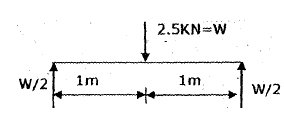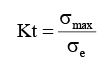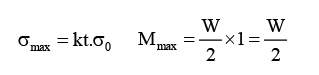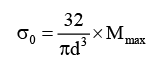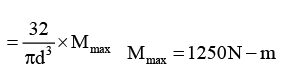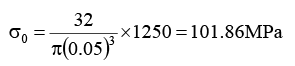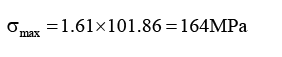QUESTION: 2

Solution:
QUESTION: 3

### Determine the length of the flat key (in mm), which is fitted on 80 mm diameter shaft, and the crosssection of the key is 16 × 10 mm. the key is transmitting 500 N-m torque from the shaft to the hub. Assume factor of safety is 3 and is made of commercial steel ( σ yt= σ yc = 230 MPa ) . Use distortion energy theory.

Solution:
QUESTION: 4

An automotive plate clutch consists of two pairs of contracting surfaces with an asbestos friction lining. The torque transmitting capacity of the clutch is 600 N-m. The permissible intensity of the pressure is 1 MPa, coefficient of friction is 0.3, and outer diameter of friction disc is 300 mm. Determine the inner diameter of the friction disc using uniform wear theory.

Solution:
QUESTION: 5

Car mass is 1400 kg and assume 65% of the car weight is loaded on to the front axle during rapid breaking. A car undergoes with an emergency stop form 96 km/hr. Determine the energy absorbed by one brake.

Solution:

W = mg

Wfront = 0.65 ×1400 × 9.81

Wfront = 8927 N

Each wheel carrying weight is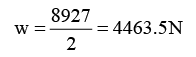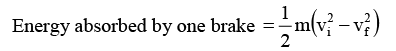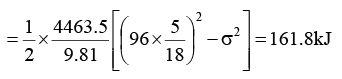QUESTION: 6

The body is subjected to three mutually perpendicular stresses, orthogonal to each other as shown in the figure. Determine the strain energy for producing only volume change. Take E = 207 GPa, ∪ = 0.25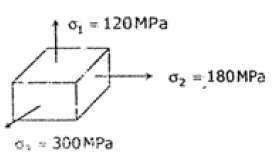Solution: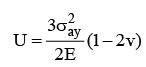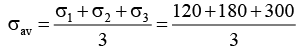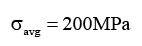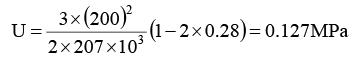QUESTION: 7

Distortion – energy theory is also called as

Solution:
QUESTION: 8

A fluctuating stress of maximum and minimum values 130 MPa and 70 MPa respectively acting on a bar. The notch sensitivity factor is 1.29. The ultimate and yield strength of the materials are 670 MPa and 480 MPa respectively. Determine the amplitude ratio.

Solution: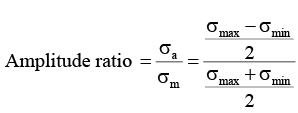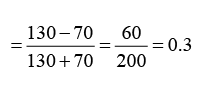QUESTION: 9

A square threaded power screw has a major diameter of 32 mm and a pitch of 4 mm with double threads. The force acting per screw is 6.4 kN. Take f = f c = 0.08 & d c = 40 mm, ‘C’ means collar. Determine the torque required to raise & lower the load.

Solution: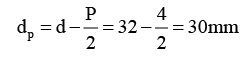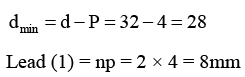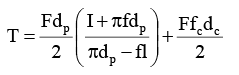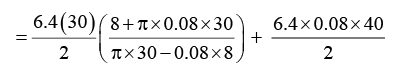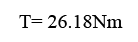QUESTION: 10

A 0.373 m2 area of bolt is subjected to a load of 6 kN in a tension joint. The initial bolt tension is 25 kN. The bolt & joint stiffness are 6.5 N/m & 13.8 N/m respectively determine the service load stresses in the bolt.

Solution: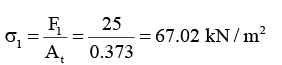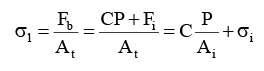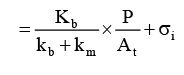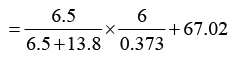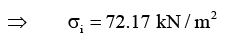QUESTION: 11

For the double long shoe, external drum brake illustrated in the figure (All dimensions are in mm), the face width of the shoes is 30 mm. the coefficient of friction between the shoe and the drum can be taken as 0.28.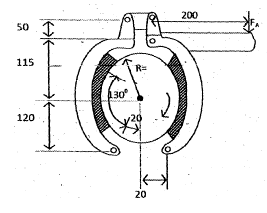Q.

Determine the torque contribution from the left-hand shoe

Solution:
QUESTION: 12

The hydro-state thrust bearing consists of six pads; each pad can be approximated as circular area of 500 mm and 100 mm as outer and inner diameters respectively. The total thrust load is 900 kN and film thickness is 0.05 mm and the viscosity of the lubricant is 58.86 cp.

Q.

Determine the supply pressure

Solution:
QUESTION: 13

The hydro-state thrust bearing consists of six pads; each pad can be approximated as circular area of 500 mm and 100 mm as outer and inner diameters respectively. The total thrust load is 900 kN and film thickness is 0.05 mm and the viscosity of the lubricant is 58.86 cp.

Q.

Determine the total flow requirement

Solution:
QUESTION: 14

Match the following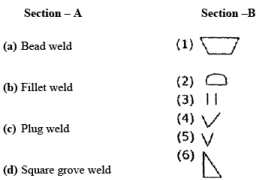Solution:
QUESTION: 15

A transmission shaft is subjected to a twisting moment of 144 kN-m whose length is 4 m the permissible angle of twist is 0.3° per meter length. The modulus of rigidity for the shaft material is 79.3 GPa. Calculate the shaft diameter.

Solution: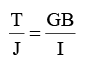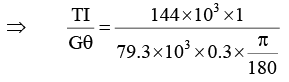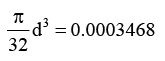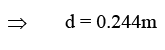QUESTION: 16

A bolt of length 60 mm and a diameter 20 mm is used to fasten two plates. The grip length of the bolt is 40 mm and the length of the unthreaded portion is 20 mm.The tensile stress area and Young’s modulus of the material are 160 mm2 and 207 GPa. Determine the stiffness of the bolt.

Solution: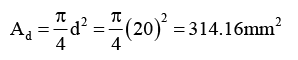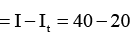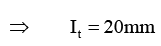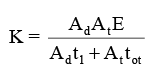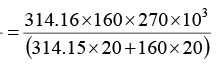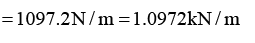QUESTION: 17

An angular contact ball bearing has a basic static load rating as 2400 kN and basic load rating is 3800 N. The ratio of axial force to basic static load rating is 0.09 & the radial load is 250 N. Determine the L10 tube at a speed of 1000 rpm. Take x = 0.56 & y = 1.527.

Solution:

Fe = XVF = yFa Fa = 0.09 × C0

Fe = 0.56 ×1× 250 + 1.527 × 216 = 469.83N Fe = 0.09 × C0 = 0.09 × 2400 = 216N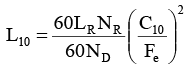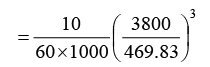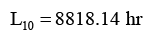QUESTION: 18

A differential band brake shown in the figure has a width & thickness of the band as 150 mm and 5 mm respectively and the permissible tensile stress is limited to 80 MPa. Coefficient of friction between friction lining & brake drum is 0.25. Determine the torque of the brake. (All dimensions are in mm)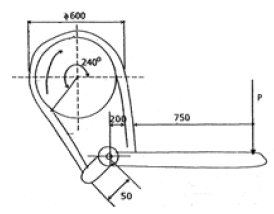Solution: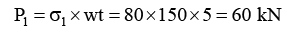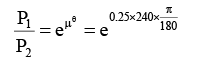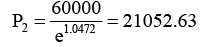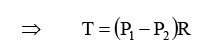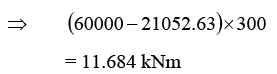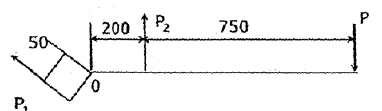QUESTION: 19

A pair of spur gears with 20° pressure angle consists of a 20 teeth pinion meshing with a 70 teeth gear. The module & face width are 3 and 30 mm respectively. The gears are heat treated to a surface hardness of 250 BHN. The maximum power transmitting capacity is __________ N.

Solution: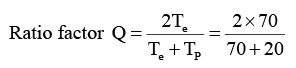Q = 1.56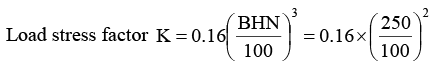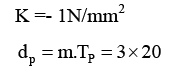⇒ 60mm

Wear strength of pinion teeth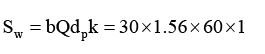= 2800N
Maximum power transmitting capacity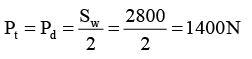QUESTION: 20

A clutch plate consists of a pair of contacting surfaces. The inner & outer diameter of the friction disks are 150 mm & 300 mm respectively. The coefficient of friction is 0.25 and the intensity of pressure is 2 MPa. Determine the frictional torque required using the uniform pressure criterion.

Solution: